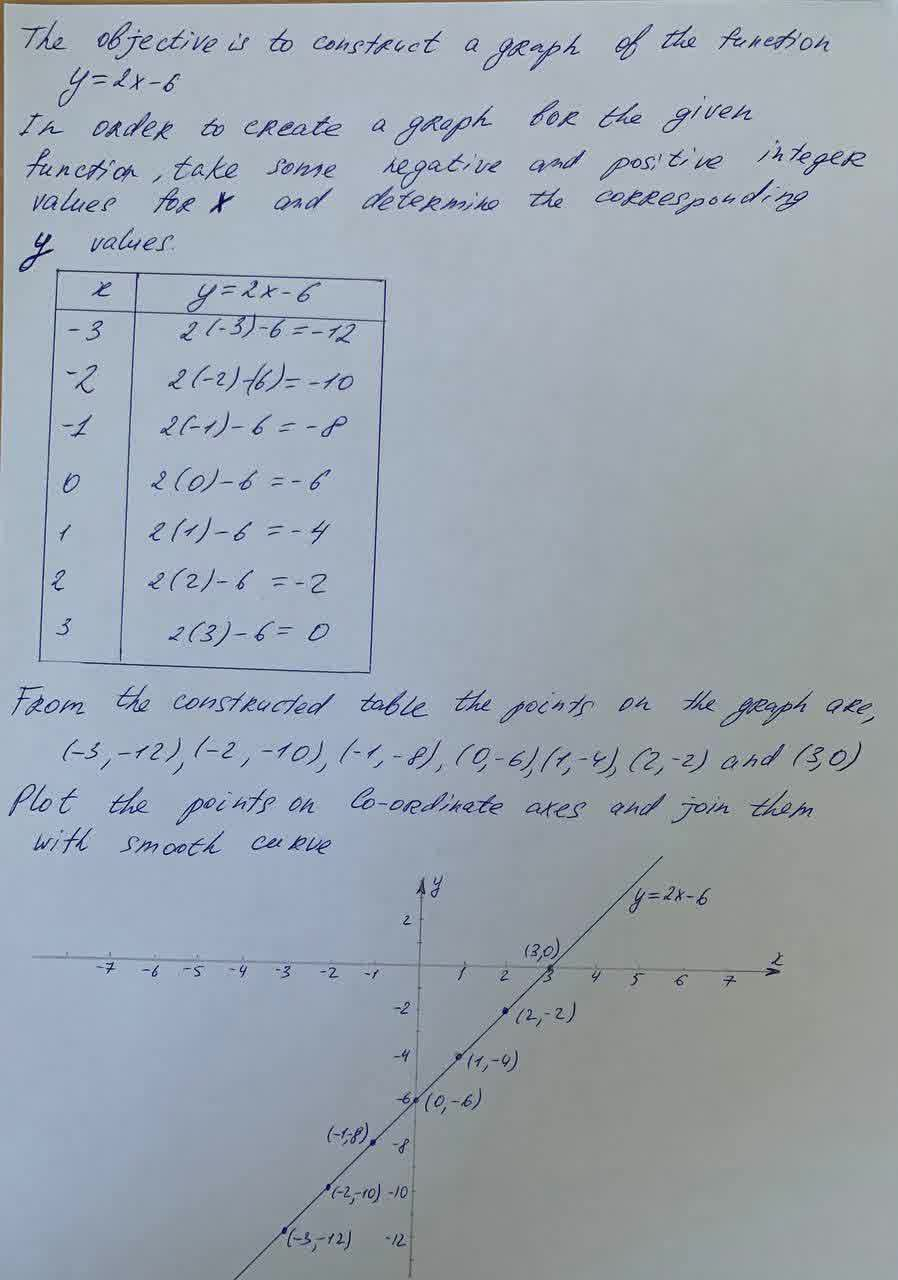# Question# Create a graph of y=2x−6. Construct a graph corresponding to the linear equation y=2x−6.

Linear equations and graphs
ANSWERED